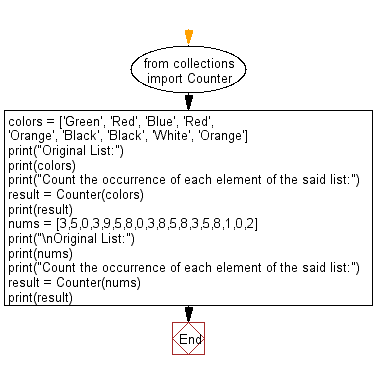﻿ Python: Count the occurrence of each element of a given list - w3resource# Python: Count the occurrence of each element of a given list

## Python Collections: Exercise-30 with Solution

Write a Python program to count the occurrence of each element of a given list.

Sample Solution:

Python Code:

``````from collections import Counter
colors = ['Green', 'Red', 'Blue', 'Red', 'Orange', 'Black', 'Black', 'White', 'Orange']
print("Original List:")
print(colors)
print("Count the occurrence of each element of the said list:")
result = Counter(colors)
print(result)
nums = [3,5,0,3,9,5,8,0,3,8,5,8,3,5,8,1,0,2]
print("\nOriginal List:")
print(nums)
print("Count the occurrence of each element of the said list:")
result = Counter(nums)
print(result)
```
```

Sample Output:

```Original List:
['Green', 'Red', 'Blue', 'Red', 'Orange', 'Black', 'Black', 'White', 'Orange']
Count the occurrence of each element of the said list:
Counter({'Red': 2, 'Orange': 2, 'Black': 2, 'Green': 1, 'Blue': 1, 'White': 1})

Original List:
[3, 5, 0, 3, 9, 5, 8, 0, 3, 8, 5, 8, 3, 5, 8, 1, 0, 2]
Count the occurrence of each element of the said list:
Counter({3: 4, 5: 4, 8: 4, 0: 3, 9: 1, 1: 1, 2: 1})
```

Flowchart:## Visualize Python code execution:

The following tool visualize what the computer is doing step-by-step as it executes the said program:

Python Code Editor:

Have another way to solve this solution? Contribute your code (and comments) through Disqus.

What is the difficulty level of this exercise?

Test your Programming skills with w3resource's quiz.

﻿

## Python: Tips of the Day

The Zip() Function:

```>>> students = ('John', 'Mary', 'Mike')
>>> ages = (15, 17, 16)
>>> scores = (90, 88, 82, 17, 14)
>>> for student, age, score in zip(students, ages, scores):
...     print(f'{student}, age: {age}, score: {score}')
...
John, age: 15, score: 90
Mary, age: 17, score: 88
Mike, age: 16, score: 82
>>> zipped = zip(students, ages, scores)
>>> a, b, c = zip(*zipped)
>>> print(b)
(15, 17, 16)
```